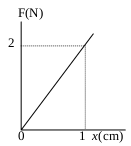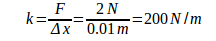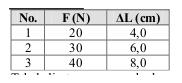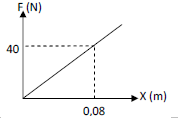# Potential energy – problems and solutions

Gravitational potential energy

1. Energy 4900 Joule used to raise an object with mass of 50 kg to a height of h. What is the height of h? Acceleration due to gravity (g) = 9.8 ms-2.

Known :

Change of potential energy (ΔPE) = 4900 Joule

Mass of object (m) = 50 kg

Acceleration due to gravity (g) = 9.8 m/s2

Wanted: height (Δh)

Solution :

ΔPE = m g Δh

4900 = (50)(9.8) Δh

4900 = 490 Δh

Δh = 10 meters

[irp]

Spring potential energy

2. The graph below shows the relation between force (F) and x (the change in length) of a spring. If the change in length of a spring is 8 cm, what is the spring potential energy?

Known :

Force (F) = 2 NewtonThe change in length 1 (x) = 1 cm = 1/100 m = 0.01 m

The change in length 2 = 8 cm = 8/100 m = 0.08 m

Wanted : The spring potential energy

Solution :

Spring’s constant :The spring potential energy :

PE = 1/2 k x2

PE = 1/2 (200 N/m)(0.08 m)2

PE = (100 N/m)(0.0064 m2)

PE = 0.64 Nm

[irp]

3. Based on table below, F = weight of object, ∆L = the change in length of spring. What is the work done on the spring so the change in length of spring is 10 cm.

Known :The change in length of spring (∆L) = 10 cm = 0.1 m

Wanted : Work done on the spring

Solution :

Spring’s constant :

k = F / ∆x = 20 N / 0.04 m = 500 N/m

k = F / ∆x = 30 N / 0.06 m = 500 N/m

k = F / ∆x = 40 N / 0.08 m = 500 N/m

Spring’s constant is 500 N/m

The work done on the spring so the change in length of spring is 10 cm :

W = 1/2 k ∆L2 = 1/2 (500 N/m)(0.1 m)2 = (250 N/m)(0.01 m2) = 2.5 N m = 2.5 Joule.

[irp]

4. Graph below shows relation between force (F) and the change in length (x). What is the spring potential energy based on graph below.

Known :F = 40 Newton

Δx = 0.08 m

Wanted : spring potential energy

Solution :

Spring’s constant :

k = F / Δx

The spring potential energy :

PE = ½ k Δx2 = ½ (F/Δx) Δx2 = ½ F Δx

PE = ½ (40)(0.08) = (20)(0.08) = 1.6 Joule

[irp]